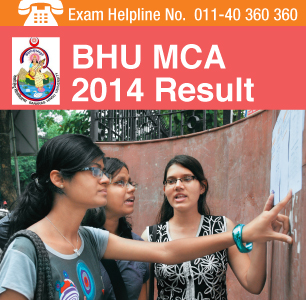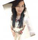BHU MCA 2014 Result is declared in online mode by the Banaras Hindu University. The result for university level BHU Post Graduate Entrance Test 2014 can be downloaded from the same page by entering CET roll number.

For any doubts and queries students can call the BHU MCA Exam2014 Helpline at 011-40360360
BHU MCA 2014 Result- Check Here

Marks obtained by the candidate in the entrance test and their overall merit and merit within the applicable class will be emailed at their registered email address. The same will also be sent through SMS at their registered mobile number provided by them in the application form. Therefore the candidates are advised to give correct e-mail addresses/mobile phone numbers for correspondence.

To settle inter se merit of candidates, case when two or more candidates secure equal marks in the entrance test, the below mentioned criteria will be adopted-

• Candidate having Mathematics at Bachelor’s level shall be considered first.
• Candidate, who has higher percentage of marks in Mathematics at Bachelor’s level, shall be considered then.
• If the tie still persists, candidate who has higher percentage of marks in Mathematics at Bachelor’s level will be preferred.
• Again the tie persists, candidate who has higher percentage of marks in Mathematics at Intermediate or equivalent examination will be preferred.
• If the tie still persists, candidates having Mathematics at Intermediate or equivalent examination, will be preferred.
• Lastly, candidate senior in age will be preferred.

BHU MCA 2014 was held on May 31, 2014 in offline mode. After result declaration, computerised counseling procedure will be held to offer admission for three years MCA course. Schedule for the BHU MCA 2014 counselling is yet to be notified.

Note: No scrutiny/ re-evaluation of the answer sheet/book of the test shall be allowed on any ground.

Stay connected to it.careers360.com for more news and feature articles on BHU MCA Entrance Exam 2014 Result

BHU MCA 2104 Cutoff

BHU MCA 2014 Answer Key

Questions related to BHU PET

Showing 218 out of 218 Questions
4 Views

i had PCM in 12th and completed BSc in botany and had chemistry in 1st and 2nd year of Bsc so am i eligilble for bhu pet exam for MSc in botany

Manidipa Kundu Student Expert 13th Oct, 2019hello,

for msc course in banaras hindu university eligibility criteria is students need to have a bachelors degree in relevant discipline. For M.Sc Zoology and M.Sc Botany, candidates must have also studied Chemistry at graduation level. as you have studied chemistry in b.sc course you are also eligible.

9 Views

Syllabus of bhu pet examination of physicsHello Debajyoti Maity

Score pattern of BHU PET

• BHU PET  questions are asked in MCQ Pattern.
• Each question will carry three marks.
• 120 questions are asked in the examination.
• Negative marking of one mark is there for each wrong answer.

Sections in the question paper are:

• Aptitude
• English Comprehension
• Language Proficiency
• Numerical and Quantitative Reasoning
• General Knowledge
• Current Affairs
• Analytical Abilities
• Mathematical Aptitude

Syllabus for English Comprehension and Language Proficiency

• Fill in the Blanks
• Improvement of Sentences
• Spotting the Error
• Synonyms &
• Spelling/ Detecting miss-spelt words
• One word Substitution
• Idioms & Phrases
• Close Passage & comprehension passage

Syllabus for Numerical and Quantitative Reasoning

• Decimals
• Exponents and Roots
• Fractions
• Integers
• Percent
• Ratio
• Real Numbers
• Coordinate Geometry
• Functions
• Graphs of Functions
• Operations with Algebraic Expressions
• Rules of Exponents
• Solving Linear Equations
• Solving Linear Inequalities
• Solving Quadratic Equations
• Circles
• Lines and Angles
• Polygons
• Three-Dimensional Figures
• Triangles
• Data Interpretation Examples
• Distributions of Data, Random Variables, and Probability Distributions
• Graphical Methods for Describing Data
• Numerical Methods for Describing Data
• Probability

Syllabus for General Knowledge and Current Affairs

• Latest Current Affairs
• Indian Constitution
• Indian History
• Indian Economy
• Indian Geography
• Indian Politics
• Indian Navy & Military

Syllabus of Mathematical Aptitude

• Fractions & Decimals,  Ratio & Proportion, Square Roots
• Simple & Compound Interest
• Time & Speed, Unitary Method,  Time & Work
• Profit & Loss
• Percentage &  Discount
• Simple Equations
• Polynomials
• Mean
• Median
• Mode
• Exponents
• Functions
• Sets
• Venn Diagrams
• Relations
• Functions
• Triangles
• Properties of Circles
• Probability
• Permutation and Combinations

Hope it helps you.

10 Views

Syllabus of bhu pet examination of physicsHello Debajyoti Maity

Score pattern of BHU PET

• BHU PET  questions are asked in MCQ Pattern.
• Each question will carry three marks.
• 120 questions are asked in the examination.
• Negative marking of one mark is there for each wrong answer.

Sections in the question paper are:

• Aptitude
• English Comprehension
• Language Proficiency
• Numerical and Quantitative Reasoning
• General Knowledge
• Current Affairs
• Analytical Abilities
• Mathematical Aptitude

Syllabus for English Comprehension and Language Proficiency

• Fill in the Blanks
• Improvement of Sentences
• Spotting the Error
• Synonyms &
• Spelling/ Detecting miss-spelt words
• One word Substitution
• Idioms & Phrases
• Close Passage & comprehension passage

Syllabus for Numerical and Quantitative Reasoning

• Decimals
• Exponents and Roots
• Fractions
• Integers
• Percent
• Ratio
• Real Numbers
• Coordinate Geometry
• Functions
• Graphs of Functions
• Operations with Algebraic Expressions
• Rules of Exponents
• Solving Linear Equations
• Solving Linear Inequalities
• Solving Quadratic Equations
• Circles
• Lines and Angles
• Polygons
• Three-Dimensional Figures
• Triangles
• Data Interpretation Examples
• Distributions of Data, Random Variables, and Probability Distributions
• Graphical Methods for Describing Data
• Numerical Methods for Describing Data
• Probability

Syllabus for General Knowledge and Current Affairs

• Latest Current Affairs
• Indian Constitution
• Indian History
• Indian Economy
• Indian Geography
• Indian Politics
• Indian Navy & Military

Syllabus of Mathematical Aptitude

• Fractions & Decimals,  Ratio & Proportion, Square Roots
• Simple & Compound Interest
• Time & Speed, Unitary Method,  Time & Work
• Profit & Loss
• Percentage &  Discount
• Simple Equations
• Polynomials
• Mean
• Median
• Mode
• Exponents
• Functions
• Sets
• Venn Diagrams
• Relations
• Functions
• Triangles
• Properties of Circles
• Probability
• Permutation and Combinations

Hope it helps you.

10 Views

Syllabus of bhu pet examination of physics

Ronak Gala Student Expert 9th Oct, 2019The following table shows the important topics of each and every subject that can be asked in the entrance test:

Section Topic
English Comprehension Fill in the Blanks
Improvement of Sentences
Spotting the Error
Synonyms & Antonyms
Spelling/ Detecting miss-spelt words
One word Substitution
Idioms & Phrases
Close Passage & comprehension passage
Quantitative Reasoning Decimals
Exponents and Roots
Fractions
Integers
Percent
Ratio
Real Numbers
Coordinate Geometry
Functions
Graphs of Functions
Operations with Algebraic Expressions
Rules of Exponents
Solving Linear Equations
Solving Linear Inequalities
Circles
Lines and Angles
Polygons
Three-Dimensional Figures
Triangles
Data Interpretation Examples
Distributions of Data, Random Variables, and Probability Distributions
Graphical Methods for Describing Data
Numerical Methods for Describing Data
Probability
General Knowledge Latest Current Affairs
Indian Constitution
Indian History
Indian Economy
Indian Geography
Indian Politics
Indian Navy & Military
Mathematical Aptitude Fractions & Decimals, Ratio & Proportion, Square Roots
Simple & Compound Interest
Time & Speed, Unitary Method, Time & Work
Profit & Loss
Percentage & Discount
Simple Equations
Polynomials
Exponents
Functions
Sets
Venn Diagrams
Relations
Functions
Triangles
Properties of Circles
Mean
Median
Mode
Probability
Permutation and Combinations
7 Views

Syllabus of bhu pet examination of physicsHello Debajyoti Maity

Score pattern of BHU PET

• BHU PET  questions are asked in MCQ Pattern.
• Each question will carry three marks.
• 120 questions are asked in the examination.
• Negative marking of one mark is there for each wrong answer.

Sections in the question paper are:

• Aptitude
• English Comprehension
• Language Proficiency
• Numerical and Quantitative Reasoning
• General Knowledge
• Current Affairs
• Analytical Abilities
• Mathematical Aptitude

Syllabus for English Comprehension and Language Proficiency

• Fill in the Blanks
• Improvement of Sentences
• Spotting the Error
• Synonyms &
• Spelling/ Detecting miss-spelt words
• One word Substitution
• Idioms & Phrases
• Close Passage & comprehension passage

Syllabus for Numerical and Quantitative Reasoning

• Decimals
• Exponents and Roots
• Fractions
• Integers
• Percent
• Ratio
• Real Numbers
• Coordinate Geometry
• Functions
• Graphs of Functions
• Operations with Algebraic Expressions
• Rules of Exponents
• Solving Linear Equations
• Solving Linear Inequalities
• Solving Quadratic Equations
• Circles
• Lines and Angles
• Polygons
• Three-Dimensional Figures
• Triangles
• Data Interpretation Examples
• Distributions of Data, Random Variables, and Probability Distributions
• Graphical Methods for Describing Data
• Numerical Methods for Describing Data
• Probability

Syllabus for General Knowledge and Current Affairs

• Latest Current Affairs
• Indian Constitution
• Indian History
• Indian Economy
• Indian Geography
• Indian Politics
• Indian Navy & Military

Syllabus of Mathematical Aptitude

• Fractions & Decimals,  Ratio & Proportion, Square Roots
• Simple & Compound Interest
• Time & Speed, Unitary Method,  Time & Work
• Profit & Loss
• Percentage &  Discount
• Simple Equations
• Polynomials
• Mean
• Median
• Mode
• Exponents
• Functions
• Sets
• Venn Diagrams
• Relations
• Functions
• Triangles
• Properties of Circles
• Probability
• Permutation and Combinations

Hope it helps you.

Top
The question have been saved in answer later, you can access it from your profile anytime. Access now